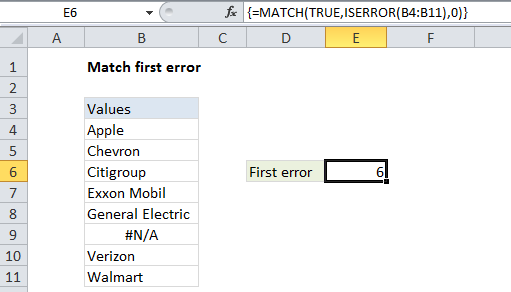Match first error in Excel

This tutorial shows how to Match first error in Excel using the example below;

Formula

`{=MATCH(TRUE,ISERROR(range),0)}`Explanation

If you need to match the first error in a range of cells, you can use an array formula based on the MATCH and ISERROR functions.

In the example shown, the formula is:

`{=MATCH(TRUE,ISERROR(B4:B11),0)}`

This is an array formula, and must be entered using Control + Shift + Enter (CSE).

Also See:   Lookup entire row in Excel

How this formula works

Working from the inside out, the ISERRROR function returns TRUE when a value is a recognized error, and FALSE if not.

When given a range of cells (an array of cells) ISERROR function will return an array of TRUE/FALSE results. In the example, this resulting array looks like this:

Also See:   Get column index in Excel Table

{FALSE;FALSE;FALSE;FALSE;FALSE;TRUE;FALSE;FALSE}

Note that the 6th value (which corresponds to the 6th cell in the range) is TRUE, since cell B9 contains #N/A.

The MATCH function is configured to match TRUE in exact match mode. It finds the first TRUE in the array created by ISERROR and returns the position. If no match is found, the MATCH function itself returns #N/A.

Also See:   Count cells that contain errors in Excel

Finding the first NA error

The formula above will match any error. If you want to match the first #N/A error, just substitute ISNA for ISERROR:

`{=MATCH(TRUE,ISNA(B4:B11),0)}`